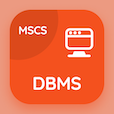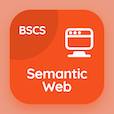Online CS Degree Courses

Digital Logic Design MCQs

Digital Logic Design MCQ - Topics

Learn Clocked Sequential Circuits Analysis Multiple Choice Questions (MCQ Quiz), Clocked Sequential Circuits Analysis quiz answers PDF to learn digital logic design course for digital logic design online classes. Synchronous Sequential Logics Multiple Choice Questions and Answers (MCQs), Clocked Sequential Circuits Analysis quiz questions for accelerated computer science degree online. Clocked Sequential Circuits Analysis Book PDF: clocked sequential circuits in dld, state reduction and assignment, flip-flops excitation tables, flip-flops in synchronous sequential logic test prep for computer and information science.

"In Moore models, the output is the function of only" MCQ PDF: clocked sequential circuits analysis App APK with present state, input state, next state, and mid state choices for accelerated computer science degree online. Study clocked sequential circuits analysis quiz questions for merit scholarship test and certificate programs for bachelor's degree in computer science.

## MCQs on Clocked Sequential Circuits Analysis Quiz

MCQ: In Moore models, the output is the function of only

present state
input state
next state
mid state

MCQ: In Mealy models output are the functions of both

present state
input state
next state
both a and b

MCQ: The time sequence for flip-flop can be enumerated by

state table
map
truth table
graph

MCQ: Latches developed with NOR and NAND gate tend to stay in the latched condition because of which configuration feature?

low input voltages
synchronous operation
gate impedance
cross coupling

MCQ: The state diagram provides the same information as the

flip-flops provides
State table provides
truth table provides
latches

### More Quizzes on Digital Logic Design Book